# DensityPage 1

#### WATCH ALL SLIDES

Slide 1## Density

Q) Which weighs more:-

A kilogram of feathers or a kilogram of iron?

Slide 2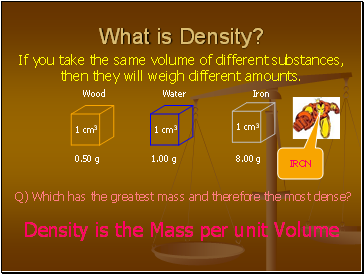## What is Density?

Density is the Mass per unit Volume

Wood

Water

Iron

1 cm3

1 cm3

1 cm3

If you take the same volume of different substances, then they will weigh different amounts.

0.50 g

1.00 g

8.00 g

Q) Which has the greatest mass and therefore the most dense?

IRON

Slide 3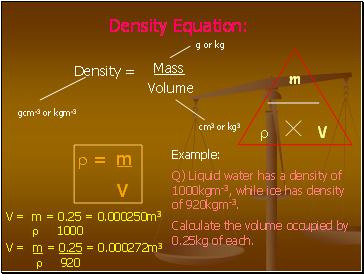## Density Equation

Density =

Mass

Volume

g or kg

cm3 or kg3

gcm-3 or kgm-3

= m

V

Example:

Q) Liquid water has a density of 1000kgm-3, while ice has density of 920kgm-3.

Calculate the volume occupied by 0.25kg of each.

:

V = m = 0.25 = 0.000250m3

 1000

V = m = 0.25 = 0.000272m3

 920

Slide 4## Density of a regular solid

Find the Mass of the solid on a balance.

Measure the three lengths and calculate the Volume.

(ie V = l x w x h )

Calculate the Density.

4.0 cm

2.0 cm

3.0 cm

 = m = 240 =10.0 g/cm3

V 24

m = 240 g

Slide 5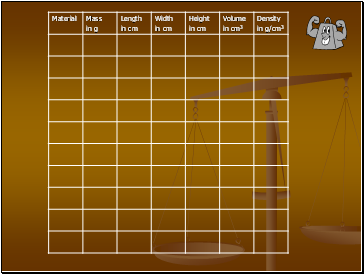Slide 6Slide 7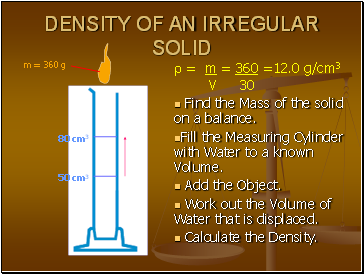DENSITY OF AN IRREGULAR SOLID

Find the Mass of the solid on a balance.

Fill the Measuring Cylinder with Water to a known Volume.

Work out the Volume of Water that is displaced.

Calculate the Density.

50 cm3

80 cm3

m = 360 g

 = m = 360 =12.0 g/cm3

V 30

Slide 8DENSITY OF AN IRREGULAR SOLID

OR use a Eureka Can to find the Volume.

Find the mass of the solid on a balance.

Place a Measuring Cylinder under the spout.

Collect the Water and read off the Volume.

Calculate Density

m = 440 g

40.0 cm3

 = m = 440 =11.0 g/cm3

V 40

Slide 9Slide 10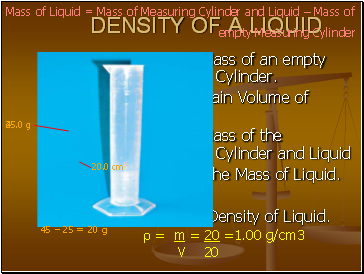## Density of a liquid

Find the Mass of an empty Measuring Cylinder.

Add a certain Volume of Liquid.

Find the Mass of the Measuring Cylinder and Liquid

Calculate the Mass of Liquid.

How?

Calculate Density of Liquid.

Go to page:
1  2